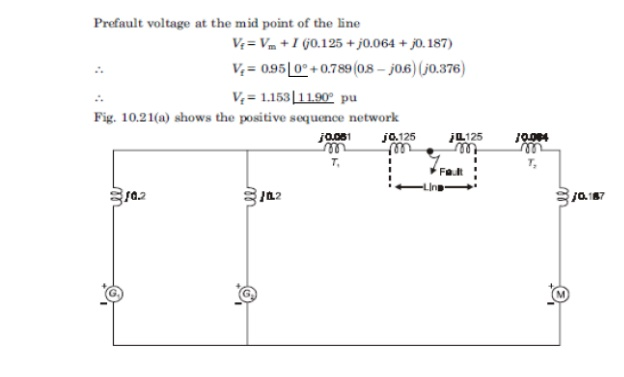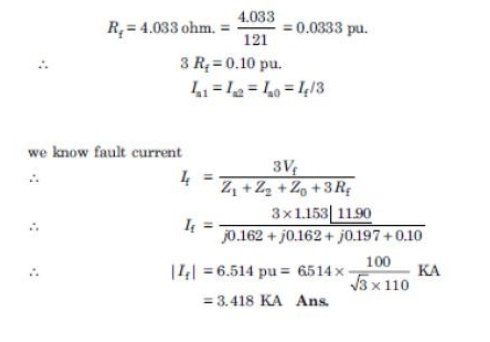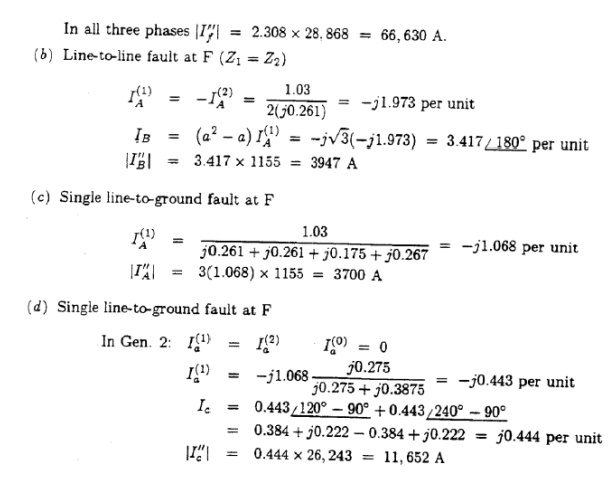Home | | Power System Analysis | Unbalanced Fault Analysis Problem Formulation

# Unbalanced Fault Analysis Problem Formulation

A 30 MVA, 13.2KV synchronous generator has a solidly grounded neutral. Its positive, negative and zero sequence impedances are 0.30, 0.40 and 0.05 p.u respectively.

Unbalanced Fault Analysis Problem Formulation

A 30 MVA, 13.2KV synchronous generator has a solidly grounded neutral. Its positive, negative and zero sequence impedances are 0.30, 0.40 and 0.05 p.u respectively. Determine the following:

a)           What value of reactance must be placed in the generator neutral so that the fault current for a line to ground fault of zero fault impedance shall not exceed the rated line current?

b)          What value of resistance in the neutral will serve the same purpose?

c)           What value of reactance must be placed in the neutral of the generator to restrict the fault current to ground to rated line current for a double line to ground fault?

d)          What will be the magnitudes of the line currents when the ground current is restricted as above?

e)           As the reactance in the neutral is indefinitely increased, what are the limiting values of the line currents?Two alternators are operating in parallel and supplying a synchronous motor which is receiving 60MW power at 0.8 power factor lagging at 6.0 KV. Single line diagram for this system is given in fig. Data are given below. Compute the fault current when a single line to ground fault occurs at the middle of the line through a fault resistance of 4.033 ohm.Study Material, Lecturing Notes, Assignment, Reference, Wiki description explanation, brief detail
Power System Analysis : Symmetrical Components And Unbalanced Fault Analysis : Unbalanced Fault Analysis Problem Formulation |